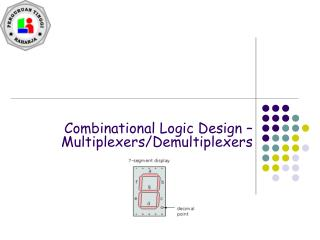DownloadDownload PresentationCombinational Logic Design – Multiplexers/Demultiplexers

# Combinational Logic Design – Multiplexers/Demultiplexers

Download Presentation## Combinational Logic Design – Multiplexers/Demultiplexers

- - - - - - - - - - - - - - - - - - - - - - - - - - - E N D - - - - - - - - - - - - - - - - - - - - - - - - - - -
##### Presentation Transcript

1. Combinational Logic Design – Multiplexers/Demultiplexers

2. Overview • Multiplexers (MUXs) • Functionality • Circuit implementation with MUXs • Demultiplexers asep@stmik-raharja.com

3. Multiplexer • “Selects” binary information from one of many input lines and directs it to a single output line. • Also known as the “selector” circuit, • Selection is controlled by a particular set of inputs lines whose # depends on the # of the data input lines. • For a 2n-to-1 multiplexer, there are 2n data input lines and n selection lines whose bit combination determines which input is selected. asep@stmik-raharja.com

4. MUX Enable 2n Data Inputs Data Output n Input Select asep@stmik-raharja.com

5. Remember the 2 – 4 Decoder? Sel(3) S1 Sel(2) Sel(1) S0 Sel(0) Mutually Exclusive (Only one O/P asserted at any time asep@stmik-raharja.com

6. 4 to 1 MUX DataFlow D3:D0 Dout 4 Control 4 2 - 4 Decoder Sel(3:0) 2 S1:S0 asep@stmik-raharja.com

7. 4-to-1 MUX (Gate level) Control Section Three of these signal inputs will always be 0. The other will depend on the data value selected asep@stmik-raharja.com

8. Multiplexer (cont.) • Until now, we have examined single-bit data selected by a MUX. What if we want to select m-bit data/words? Combine MUX blocks in parallel with common select and enable signals • Example: Construct a logic circuit that selects between 2 sets of 4-bit inputs (see next slide for solution). asep@stmik-raharja.com

9. Example: Quad 2-to-1 MUX • Uses four 4-to-1 MUXs with common select (S) and enable (E). • Select line chooses between Ai’s and Bi’s. The selected four-wire digital signal is sent to the Yi’s • Enable line turns MUX on and off (E=1 is on). asep@stmik-raharja.com

10. Implementing Boolean functions with Multiplexers • Any Boolean function of n variables can be implemented using a 2n-1-to-1 multiplexer. A MUX is basically a decoder with outputs ORed together, hence this isn’t surprising. • The SELECT signals generate the minterms of the function. • The data inputs identify which minterms are to be combined with an OR. asep@stmik-raharja.com

11. Example • F(X,Y,Z) = X’Y’Z + X’YZ’ + XYZ’ + XYZ = Σm(1,2,6,7) • There are n=3 inputs, thus we need a 22-to-1 MUX • The first n-1 (=2) inputs serve as the selection lines asep@stmik-raharja.com

12. Efficient Method for implementing Boolean functions • For an n-variable function (e.g., f(A,B,C,D)): • Need a 2n-1 line MUX with n-1 select lines. • Enumerate function as a truth table with consistent ordering of variables (e.g., A,B,C,D) • Attach the most significant n-1 variables to the n-1 select lines (e.g., A,B,C) • Examine pairs of adjacent rows (only the least significant variable differs, e.g., D=0 and D=1). • Determine whether the function output for the (A,B,C,0) and (A,B,C,1) combination is (0,0), (0,1), (1,0), or (1,1). • Attach 0, D, D’, or 1 to the data input corresponding to (A,B,C) respectively. asep@stmik-raharja.com

13. Another Example • Consider F(A,B,C) = m(1,3,5,6). We can implement this function using a 4-to-1 MUX as follows. • The index is ABC. Apply A and B to the S1 and S0 selection inputs of the MUX (A is most sig, S1 is most sig.) • Enumerate function in a truth table. asep@stmik-raharja.com

14. MUX Example (cont.) When A=B=0, F=C When A=0, B=1, F=C When A=1, B=0, F=C When A=B=1, F=C’ asep@stmik-raharja.com

15. MUX implementation of F(A,B,C) = m(1,3,5,6) A B C C F C C’ asep@stmik-raharja.com

16. Or Simply…. C C 11 10 01 00 F C C A B asep@stmik-raharja.com

17. A larger Example asep@stmik-raharja.com

18. MUX as a Universal Gate • We can construct OR, AND, and NOT gates using 2-to-1 MUXs. Thus, 2-to-1 MUX is a universal gate. NOT AND OR 1 x1 z = x1+ x1’x0 = x1x0’ + x1x0 + x1’x0 = x1 + x0 z = 0x + 1x’ = x’ z = x1x0 + 0x0’ = x1x0 asep@stmik-raharja.com

19. Demultiplexers (DMUX) • Performs the inverse of a multiplexing operation: • Receives data from a single line • Transmit it to one of the 2n possible output lines • Selection of a specific output is controlled by the n select lines • Demultiplexers are basically decoders! For example, a 2-to-4 DMUX is a 2-to-4 decoder with enable input. asep@stmik-raharja.com